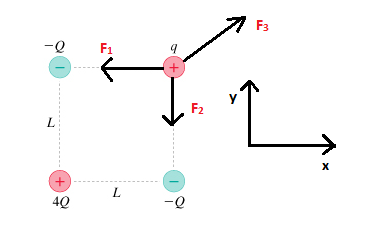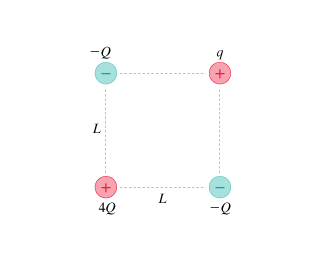# Problem: The figure shows four charges at the corners of a square of side L. Assume q and Q are positive.What is the magnitude of the net force on q?Give your answer in terms of Q, q, L and constant K.

###### FREE Expert Solution

Coulomb's law:

$\overline{){\mathbf{F}}{\mathbf{=}}\frac{\mathbf{k}{\mathbf{Q}}_{\mathbf{1}}{\mathbf{Q}}_{\mathbf{2}}}{{\mathbf{r}}^{\mathbf{2}}}}$F1 = -kQq/L2 i

F2 = -kQq/L2 j

(r3)2 = (2L2)

93% (367 ratings)###### Problem Details

The figure shows four charges at the corners of a square of side L. Assume q and Q are positive.

What is the magnitude of the net force on q?

Give your answer in terms of Q, q, L and constant K.